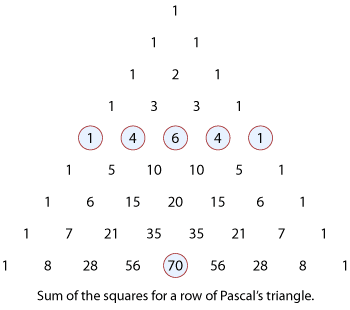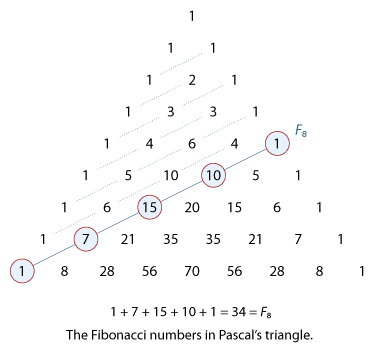## Content

### Further identities and results

#### Sum of the squares of the binomial coefficients

In the following diagram of Pascal's triangle, we see that by summing the squares of the

numbers in the fourth row,

$1^2+ 4^2+6^2+4^2+1^2=70,$

we get the middle number of the eighth row.Detailed description of diagram

We now explain the general pattern.

Using the fact that

$\dbinom{n}{r} = \dbinom{n}{n-r},$

we can write the binomial expansion in two different ways:

\begin{align*} (1+x)^n &= \dbinom{n}{0}+ \dbinom{n}{1}x + \dbinom{n}{2}x^2+\dots+\dbinom{n}{r}x^r+\dots+ \dbinom{n}{n-1}x^{n-1} + \dbinom{n}{n}x^n,\\ (1+x)^n &= \dbinom{n}{n}+ \dbinom{n}{n-1}x + \dbinom{n}{n-2}x^2+\dots+\dbinom{n}{r}x^{n-r}+\dots+ \dbinom{n}{1}x^{n-1} + \dbinom{n}{0}x^n. \end{align*}

Multiplying these two expansions together, we see that in $$(1+x)^n(1+x)^n$$ the

coefficient of $$x^n$$ is

$\dbinom{n}{0}^2 + \dbinom{n}{1}^2+\dots+\dbinom{n}{r}^2+\dots+\dbinom{n}{n}^2.$

Also, since

$(1+x)^n (1+x)^n = (1+x)^{2n},$

the coefficient of $$x^n$$ in this expansion is $$\dbinom{2n}{n}$$. Equating the two expressions

for the coefficient of $$x^n$$ we have

$\dbinom{2n}{n}= \dbinom{n}{0}^2 + \dbinom{n}{1}^2+\dots+ \dbinom{n}{r}^2+\dots+\dbinom{n}{n}^2.$

Using summation notation, this identity is

$\dbinom{2n}{n}=\sum_{r=0}^{n}\dbinom{n}{r}^2.$

The case for $$n = 4$$ was given at the start of this section:

$\dbinom{8}{4} = \dbinom{4}{0}^2 + \dbinom{4}{1}^2+ \dbinom{4}{2}^2 + \dbinom{4}{3}^2+ \dbinom{4}{4}^2.$
##### Exercise 10

The number of forward paths from the bottom left to the top right of an $$n\times n$$ grid

is $$\dbinom{2n}{n}$$. Use an argument involving paths in the grid to prove

$\dbinom{2n}{n}= \dbinom{n}{0}^2 + \dbinom{n}{1}^2+\dots+ \dbinom{n}{r}^2+\dots+\dbinom{n}{n}^2.$

(Hint. Draw a diagonal line across the grid from the top-left corner to the bottom-right corner. Choose a point of the grid on the diagonal line. Consider the number of forward paths from the bottom-left corner to the point and then the number of paths from the point to the top-right corner. Repeat for each point on the diagonal line.)

#### Yet more results and identities

We give some further results that come from applying the binomial theorem to $$(1 + x)^n$$.

##### Exercise 11

Each of the following expansions has three coefficients in arithmetic progression:

\begin{align*} (1+x)^7 &= 1+7x+21x^2+35x^3+35x^4+21x^5+7x^6+x^7,\\ (1+x)^{14} &= 1+14x+91x^2+364x^3+1001x^4+2002x^5+3003x^6+3432x^7+\dotsb,\\ (1+x)^{23} &= \dotsb + 490\,314 x^8 + 817\,190 x^9 + 1\,144\,066 x^{10} + \dotsb. \end{align*}

In the first expansion, where $$n=7$$, the arithmetic progression is 7, 21, 35.

In the second expansion, where $$n=14$$, the progression is 1001, 2002, 3003.

For the expansion of $$(1+x)^n$$, where $$n>2$$, prove that if the coefficients of three

consecutive powers are in arithmetic progression, then $$n+2$$ is a perfect square.

(Hint. Consider three coefficients $$\dbinom{n}{r-1}$$, $$\dbinom{n}{r}$$, $$\dbinom{n}{r+1}$$.)

#### Example

Prove the identity

$n\times2^{n-1}= \sum_{k=0}^{n}k\times\dbinom{n}{k}$

and check in the case when $$n=4$$.

#### Solution

We begin with the expansion of $$(1 + x)^n$$ from the binomial theorem:

$(1+x)^n= \sum_{k=0}^{n}\dbinom{n}{k}x^k.$

Differentiating both sides of this identity with respect to $$x$$ gives

$n(1+x)^{n-1}= \sum_{k=0}^{n}k\dbinom{n}{k}x^{k-1}.$

Now substitute $$x=1$$ to obtain the result:

$n2^{n-1}= \sum_{k=0}^{n}k\dbinom{n}{k}.$

When $$n=4$$, the left-hand side is $$4\times2^3 = 32$$ and the right-hand side is

\begin{align*} 4+2\times\dbinom{4}{2} +3\times\dbinom{4}{3}+4 &=4+2\times6+3\times4+4\\ &=32. \end{align*}

If we substitute $$x = -1$$ into the identity from the previous example,

$n(1+x)^{n-1}= \sum_{k=0}^{n}k\dbinom{n}{k}x^{k-1},$

then we obtain

$\sum_{k=0}^{n}k\dbinom{n}{k}(-1)^{k-1} = 0.$

For example, when $$n=6$$ this gives

$\dbinom{6}{1} +3 \dbinom{6}{3} + 5\dbinom{6}{5} = 2\dbinom{6}{2} + 4\dbinom{6}{4}+ 6\dbinom{6}{6}.$

#### A link to the Fibonacci numbers

Let $$F(n)$$ denote the sum

$F(n) = \dbinom{n}{0} + \dbinom{n-1}{1} + \dbinom{n-2}{2} + \dotsb$

where we keep summing until the lower number exceeds the top one. Then

$F(n+1) = \dbinom{n+1}{0} + \dbinom{n}{1} + \dbinom{n-1}{2} + \dotsb.$

Adding the first term in line 1 with the second in line 2 and so on, we get

$F(n+1) + F(n) = \dbinom{n+1}{0} + \Bigg[\dbinom{n}{0} + \dbinom{n}{1}\Bigg] + \Bigg[\dbinom{n-1}{1} + \dbinom{n-1}{2}\Bigg] + \dotsb.$

Using Pascal's identity, we obtain

$F(n+1)+F(n) = \dbinom{n+2}{0}+ \dbinom{n+1}{1}+ \dbinom{n}{2} +\cdots = F(n+2).$

Since $$F(0)=F(1)=1$$, we have shown that $$F(0)$$, $$F(1)$$, $$F(2)$$, $$F(3)$$, $$\dots$$ is the sequence of Fibonacci numbers.

This result can be demonstrated in terms of Pascal's triangle.Detailed description of diagram

Next page - Links forward - A result using complex numbers